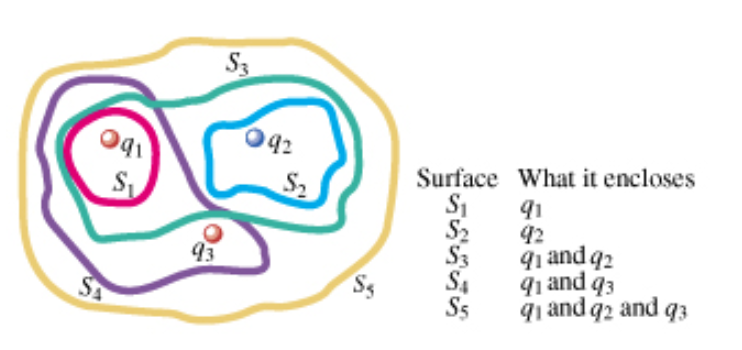# Problem: The three small spheres shown in the figure (Figure 1) carry charges q1 = 3.95 nC, q2 = -7.75 nC, and q3 = 2.50 nC.A. Find the net electric flux through the closed surface S2 shown in cross section in the figure.B. Find the net electric flux through the closed surface S3 shown in cross section in the figure.C. Find the net electric flux through the closed surface S4 shown in cross section in the figure.D. Find the net electric flux through the closed surface S5 shown in cross section in the figure.

###### FREE Expert Solution

Electric flux is given by:

$\overline{){{\mathbf{\varphi }}}_{{\mathbf{E}}}{\mathbf{=}}\frac{{\mathbf{q}}_{\mathbf{e}\mathbf{n}\mathbf{c}\mathbf{l}}}{{\mathbf{\epsilon }}_{\mathbf{0}}}}$

87% (304 ratings)###### Problem Details

The three small spheres shown in the figure (Figure 1) carry charges q1 = 3.95 nC, q2 = -7.75 nC, and q3 = 2.50 nC.A. Find the net electric flux through the closed surface S2 shown in cross section in the figure.

B. Find the net electric flux through the closed surface S3 shown in cross section in the figure.

C. Find the net electric flux through the closed surface S4 shown in cross section in the figure.

D. Find the net electric flux through the closed surface S5 shown in cross section in the figure.